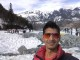# How to reverse the ids in a mysql table column without breaking primary key constraint?

Let me explain the problem scenario: Let us assume we have a table with 4 columns in it out of which 1 is `PRIMARY `column and rest 3 contain some data.

What we want to do is to reverse the primary key IDs for that data keeping the rest of data intact. It is like shifting the first row to end and moving last row to starting.

## Initial Data

 id name email anything 1 jacob whatever how to reverse 3 nathan whosoeverisnathan ids in mysql table column 4 jagmohan idontknowjagmohan without breaking 8 monica iamsexy primary key constraint 9 batman idontexistinrealworld i am batman

## Data after update

 id name email anything 9 jacob whatever how to reverse 8 nathan whosoeverisnathan ids in mysql table column 4 jagmohan idontknowjagmohan without breaking 3 monica iamsexy primary key constraint 1 batman idontexistinrealworld i am batman

## Dude, you are just reversing the column, what is so tough in this?

So, this seems simple to reverse an array if isolated by key “id“. But you have to understand that this column is the primary key. So if you run a command to change id for “jacob” to “9“. It will give you error: “`Duplicate entry for id 9`

So here is my proposed solution, I start with pair of first and last row and then swap them. Then swap second and second last row. and So on…

If total rows are odd, we will be left with 1 row which does not need correction because it will already be the middle row.

If total rows are even, we would swap middle two rows too.

Here is my solution in PHP

```\$possible_group_id="3";
\$ai=12720; //can be any high int which does not exist in column `id` yet
\$q="select id,email from table_name where `possible_group_id`=\$possible_group_id order by id asc";
\$r=mysqli_query(\$f,\$q);
\$row_collection=[];
\$ct=[];
while(\$row=mysqli_fetch_row(\$r))
{
\$row_collection[]=\$row;
\$ct[]=\$row;
}
\$pt=array_reverse(\$ct);
\$size=count(\$row_collection);
for(\$i=0;\$i<\$size;\$i++)
{
\$row_collection[\$i]=\$pt[\$i];
}
echo "start transaction;<br>";
foreach(\$row_collection as \$k=>\$v)
{
if(\$k < floor(\$size/2)){
echo 'UPDATE `table_name` SET `id`='.\$ai.' WHERE `email`="'.\$row_collection[\$size-\$k-1].'";<br>';
echo 'UPDATE `table_name` SET `id`='.\$v.' WHERE `email`="'.\$v.'";<br>';
echo 'UPDATE `table_name` SET `id`='.\$row_collection[\$k].' WHERE `email`="'.\$row_collection[\$size-\$k-1].'";<br>';}
else break;
}
echo "commit;<br>";
```

It would output this:

```start transaction;
UPDATE `table_name` SET `id`=12720 WHERE `email`="idontexistinrealworld ";
UPDATE `table_name` SET `id`=9 WHERE `email`="whatever";
UPDATE `table_name` SET `id`=1 WHERE `email`="idontexistinrealworld ";
UPDATE `table_name` SET `id`=12720 WHERE `email`="iamsexy";
UPDATE `table_name` SET `id`=8 WHERE `email`="whosoeverisnathan";
UPDATE `table_name` SET `id`=3 WHERE `email`="iamsexy";
commit;
```## Author: Harshvardhan Malpani

PHP Developer based in New Delhi, India. Working as a freelance web developer providing server deployment, website development and maintenance services.